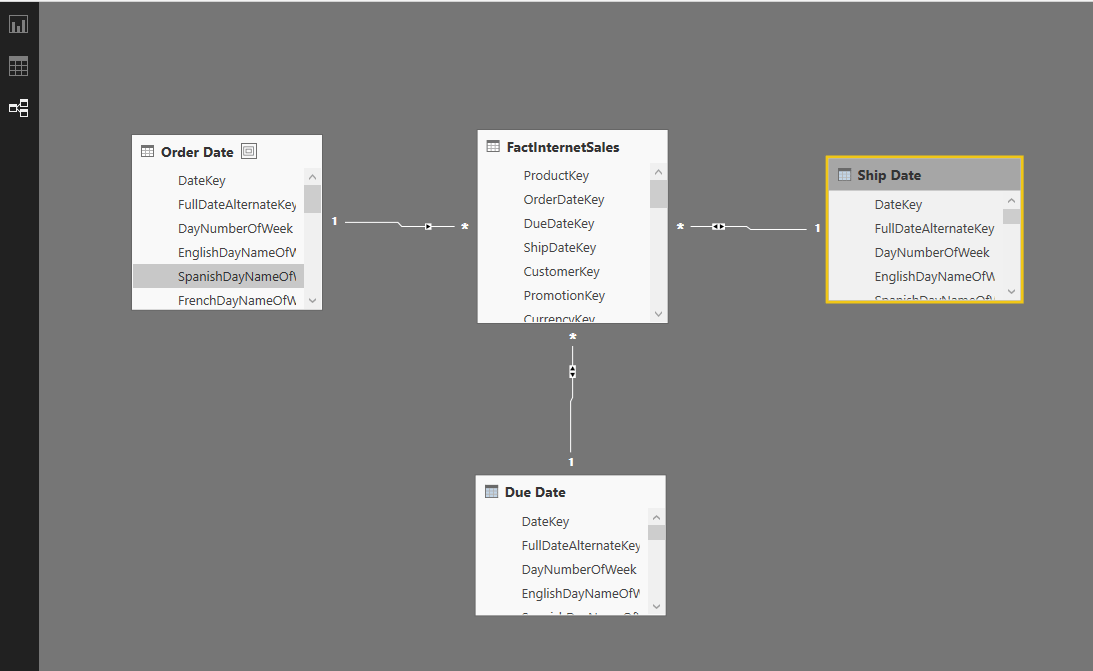# Scenarios of Using Calculated TablesPlease sign up for the course before starting the lesson.

Calculated tables first introduced in September 2015 update of Power BI Desktop. The name speak for itself; these are tables created by calculation. As these are in-memory tables their calculation is based on DAX (Data Analysis eXpression language). There are many benefits of using Calculated tables, such as using them for role playing dimensions (for example having more than one date dimension in a model), There are some DAX functions and expressions that returns a table as a result, and using them as table in your model sometimes is really helpful. for example you might want to create a table for top 10 customers and then use that as the main source table in many reports. In this lesson I’ll explain to you some use cases of calculated tables.

Back to: Power BI Essentials > Learning DAX